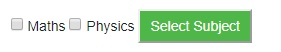# Passing Checkbox Data to CGI Program in Python

Checkboxes are used when more than one option is required to be selected.

## Example

Here is example HTML code for a form with two checkboxes −

<form action = "/cgi-bin/checkbox.cgi" method = "POST" target = "_blank">
<input type = "checkbox" name = "maths" value = "on" /> Maths
<input type = "checkbox" name = "physics" value = "on" /> Physics
<input type = "submit" value = "Select Subject" />
</form>

## Output

The result of this code is the following form −Below is checkbox.cgi script to handle input given by web browser for checkbox button.

#!/usr/bin/python
# Import modules for CGI handling
import cgi, cgitb
# Create instance of FieldStorage
form = cgi.FieldStorage()
# Get data from fields
if form.getvalue('maths'):
math_flag = "ON"
else:
math_flag = "OFF"
if form.getvalue('physics'):
physics_flag = "ON"
else:
physics_flag = "OFF"
print "Content-type:text/html\r\n\r\n"
print "<html>"
print "</html>"